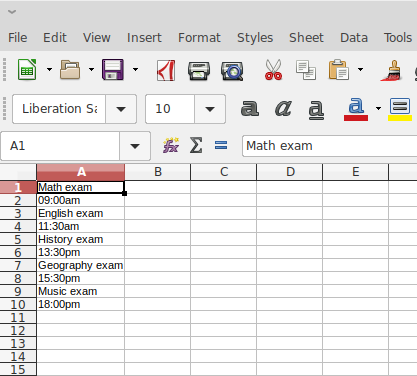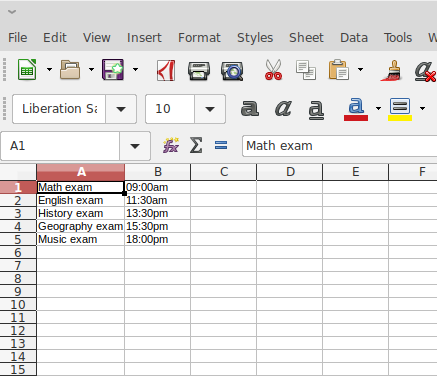# LibreOffice Calc move every nth row

Hi.

I have a text document with every line in the A column. I want to move every second cell into the B column one cell above, and then delete the empty row. Exactly how do I do this? I am not familiar with using LibreOffice Calc, so please explain it to me with detailed instructions. Thank you.

BeforeAfterHello,

another solution:

• Put the following formula to cell `B1`: `=OFFSET(\$A\$1,(COLUMN()-1)+(ROW()-1)*2-1,0)`
• Drag the formula to cell `C1` (or just copy)
• Select cells `B1:C1`
• Drag copy down to row `5` (now cells `B1` to `C5` should show what you want to achieve; attached sample file is at this stage)
• Select columns `B:C`
• Perform `Data -> Calculate -> Formula to Value`
• Delete column `A`

See the following sample file: ArrangeEveryNth.ods

Hope that helps.

If the answer is correct or helped you to solve your problem, please click the check mark () next to the answer.

This is very good. Thanks for the help. I have one additional question. What would the formula be if you had say 5 rows of data at a time that you wanted to move into adjacent columns? The same thing, but instead of two.should have pointed out where the `N` in my formula is:

``````N=2: =OFFSET(\$A\$1,(COLUMN()-1)+(ROW()-1)*2-1,0)
N=3: =OFFSET(\$A\$1,(COLUMN()-1)+(ROW()-1)*3-1,0)
N=4: =OFFSET(\$A\$1,(COLUMN()-1)+(ROW()-1)*4-1,0)
N=5: =OFFSET(\$A\$1,(COLUMN()-1)+(ROW()-1)*5-1,0)``````

Thank you so much!

Insert a further row in the beginning of the sheet. Write in A1 Content and in B1 Number.

Number column B this way: 1-2-1-2-1-2 or A-B-A-B… downwards. Do this: Insert in B2 A and in B3 B. Select cells B2 and B3 and cut the content out (CTRL+X). Then select B2 to B10 (or more) and insert clipboard. It will have the form of A-B-A-B-A… or 1-2-1-2-1-2 …

Select A1 and B1 and (Data menu) insert AutoFilter.

B1 contains filters (1 and 2 or A and B). Select 1 (or A) in filter.
Copy values of column A.
Create a new sheet and insert clipboard in A1 (or B1).
Go back to first sheet and select 2 (or B) in filter. Copy and paste into sheet 2.

Probably there’s a more elegant solution?